Courses

# Principle Of Effective Stress, Capillarity & Permeability - 2

## 10 Questions MCQ Test Mock Test Series for Civil Engineering (CE) GATE 2020 | Principle Of Effective Stress, Capillarity & Permeability - 2

Description
This mock test of Principle Of Effective Stress, Capillarity & Permeability - 2 for Civil Engineering (CE) helps you for every Civil Engineering (CE) entrance exam. This contains 10 Multiple Choice Questions for Civil Engineering (CE) Principle Of Effective Stress, Capillarity & Permeability - 2 (mcq) to study with solutions a complete question bank. The solved questions answers in this Principle Of Effective Stress, Capillarity & Permeability - 2 quiz give you a good mix of easy questions and tough questions. Civil Engineering (CE) students definitely take this Principle Of Effective Stress, Capillarity & Permeability - 2 exercise for a better result in the exam. You can find other Principle Of Effective Stress, Capillarity & Permeability - 2 extra questions, long questions & short questions for Civil Engineering (CE) on EduRev as well by searching above.
QUESTION: 1

Solution:
QUESTION: 2

### In a soil deposit of three layers of equal thickness, the permeabilities of first, second and third layers are in the ratio of 1 : 2 : 3. The ratio of average permeability in horizontal direction (kh) to that in the vertical direction (kv) will be

Solution:

Thickness of each layer is same i.e.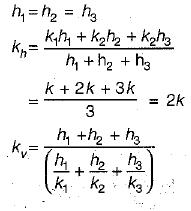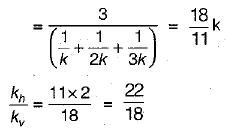QUESTION: 3

### Rise of water table above the ground surface causes:

Solution:

Water table above the ground surface acts as surcharge hence total stress also increases.

Also,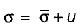and as u increases c increases

QUESTION: 4

The water level in a lake is 5 m above the bed. The saturated unit weight of the lake bed soil is 20 kN/m3. The unit weight of water is 10 kN/m3. The effective vertical stress at 5 m depth below . the lake bed is

Solution: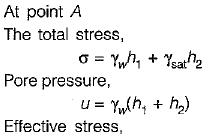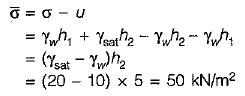QUESTION: 5

Due to rise in temperature, the viscosity and the unit weight of the percolating fluid are reduced to 60% and 90% respectively. If other things remain constant, the coefficient of permeability

Solution: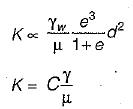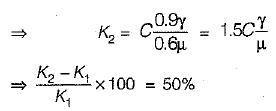QUESTION: 6

Which of these statements are correct?

Consider the following statements:

1. On dry side of optimum moisture content point the structure of soil is more of dispersed nature.

2. Activity is defined for sand particles

3. Activity is defined for clay particles

4. On dry side of optimum moisture content point the structure of soil is more of dispersed nature.

Solution:

Solution A

On the dry side of optimum moisture content point the structure of soil is more of flocculated nature.

Activity is defined for clays. Activity is the ratio of plasticity index to percentage of particles finer than 2 micron.

QUESTION: 7

Effective stress on soil:

Solution:
QUESTION: 8

In a falling-head permeability test, the time taken for the head to fall from 27 cm to 3 cm is 10 minutes, if the test is repeated with the same initial head, i.e., 27 cm, what time would it take for the head to fall to 9 cm?

Solution: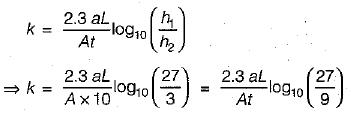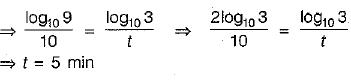QUESTION: 9

The ratio of Darcy’s coefficient of permeability to porosity is known as:

Solution: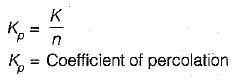QUESTION: 10

The soils most susceptible to liquefaction are

Solution:

In loose saturated sands on disturbance soil molecules came closer and volume decreases and hence positive pore pressure is set up, as a result effective stress reduces and sudden loss in shear strength is observed which results into failure. Such phenomenon is called liquefaction of sands.